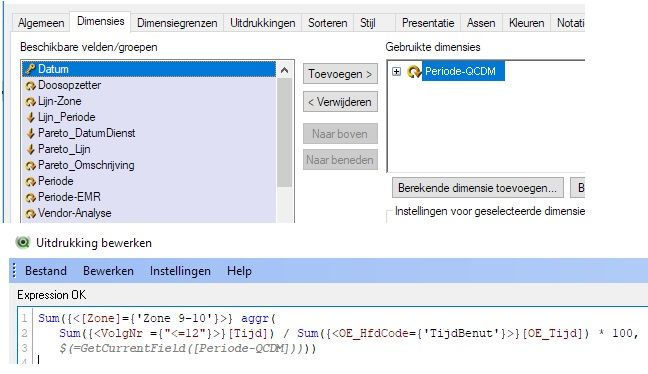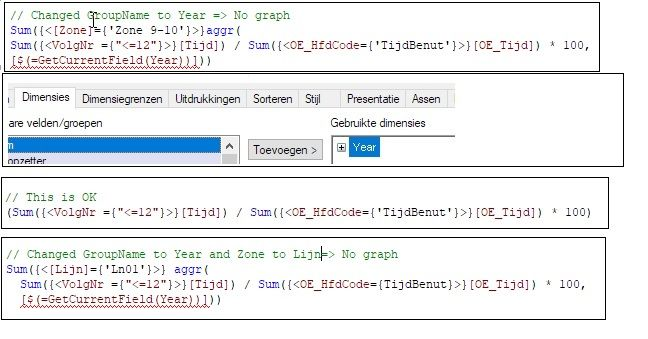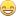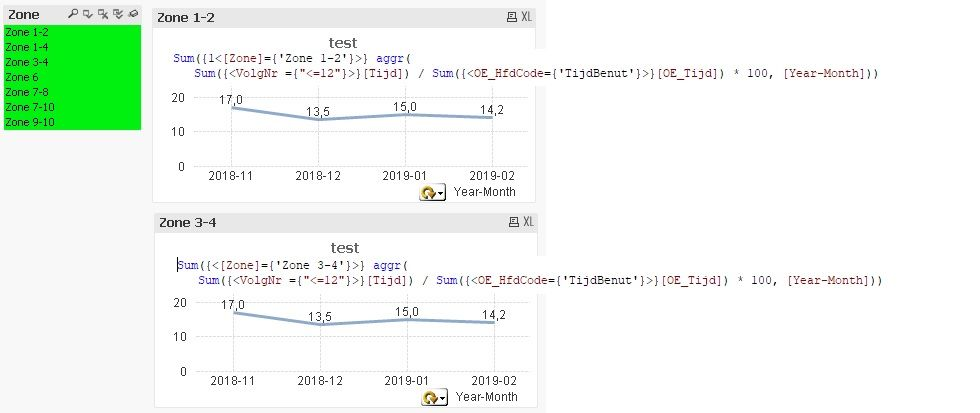# New to QlikView

Discussion board where members can get started with QlikView.

Announcements
Qlik Analytics Tour 2020 Online. Begins August 10th. Register Today
cancel
Showing results for
Did you mean:
HighlightedContributor III

## Using Variable in expression for Graph

Currently I have the below graph based on a value in the table (TIJD)

I do however want to base it on a calculated value.  For this calculated value I created a Variable :  vTL%

vTL% : (((Sum({<VolgNr ={1}>}[Tijd]) + Sum({<VolgNr ={2}>}[Tijd])+Sum({<VolgNr ={3}>}[Tijd]) + Sum({<VolgNr ={4}>}[Tijd])+Sum({<VolgNr ={5}>}[Tijd]) + Sum({<VolgNr ={6}>}[Tijd])+Sum({<VolgNr ={7}>}[Tijd])
+
Sum({<VolgNr ={8}>}[Tijd])+ Sum({<VolgNr ={9}>}[Tijd]) + Sum({<VolgNr ={10}>}[Tijd])+ Sum({<VolgNr ={11}>}[Tijd]) + Sum({<VolgNr ={12}>}[Tijd])) / Sum({<OE_HfdCode={'TijdBenut'}>}[OE_Tijd]))) * 100

Putting this in the default expresion does not work      Sum({<[Zone]={'Zone 9-10'}>} \$(vTL%)

How must I setup my expression to make this work?

Thanks.

JanLabels (3)

• ### Variables

1 Solution

Accepted Solutions
HighlightedContributor III

## Re: Using Variable in expression for Graph

Thanks for helping so far.

Jan Droog

10 Replies
HighlightedMVP & Luminary

## Re: Using Variable in expression for Graph

Your approach doesn't work because you are nesting aggregation-functions without an aggr() and this isn't valid.

To get it working it should rather look like:

Sum({<[Zone]={'Zone 9-10'}>} aggr(
Sum
({<VolgNr ={"<=12"}>}[Tijd]) / Sum({<OE_HfdCode={'TijdBenut'}>}[OE_Tijd]) * 100,
\$(=getcurrentfield('YourDimensionGroupName'))))

Here your redandant summing of the various VolgNr are shortened into a single calculation by adjusting the set analysis. The "<=12" might be extended with a >= or replaced with just a listing like 1,2,3, ... Further the needed dimension for the aggr is fetched from the used dimension-group. And the further set analysis is applied within the outer aggregation.

- Marcus

HighlightedContributor III

## Re: Using Variable in expression for Graph

I changed the expression as you proposed. Still no graph.

I first changed it to a single calculation. That worked well.

Then with the aggr(.............  Still no graph.

What's missing?

```Sum({<[Zone]={'Zone 9-10'}>} aggr(
Sum({<VolgNr ={"<=12"}>}[Tijd]) / Sum({<OE_HfdCode={'TijdBenut'}>}[OE_Tijd]) * 100,
\$(=GetCurrentField([Periode-QCDM]))))```

The GroupName is equal to the one mentioned in 'Demensions'section

HighlightedMVP & Luminary

## Re: Using Variable in expression for Graph

The dimension-group isn't treated as a classical field and needs therefore no [] brackets for itself else just type it without brackets or any quotes and ignore any syntax highlightning errors on it, but the whole construct must be wrapped with brackets if the belonging fields have some special chars or spaces. This means it should be look like:

`[\$(=GetCurrentField(Periode-QCDM))]`

If it's don't work switch your group on the Year-Month field and replace the getcurrentfield() with it - to check if the main-logic worked.

- Marcus

HighlightedContributor III

## Re: Using Variable in expression for Graph

Marcus many thanks for your input so far. Unfortunately I didn't succeed yet.

I Think I tried what you proposed me to do.

Hope I give you enough information to give it another tryHighlightedMVP & Luminary

## Re: Using Variable in expression for Graph

If you don't use the dimension-group within the chart you mustn't use getcurrentfield() else just the field like:

Sum({<[Zone]={'Zone 9-10'}>} aggr(
Sum
({<VolgNr ={"<=12"}>}[Tijd]) / Sum({<OE_HfdCode={'TijdBenut'}>}[OE_Tijd]) * 100,
Year))

- Marcus

HighlightedContributor III

## Re: Using Variable in expression for Graph

That did itWhy however does the graph still show the same data in each graph despite the different Zone in the expression.

What I want is a worksheet with 5 graphs for each zone simultaneouslyHighlightedMVP & Luminary

## Re: Using Variable in expression for Graph

Are you sure that the values behind it are really different? Especially in regard to the association of the field Zone?

Another point could be the 1 as set modifier within you first chart.

- Marcus

HighlightedContributor III

## Re: Using Variable in expression for Graph

Yes the values are different.  It's now showing the avarage of all selection Zone's and it's value changes with a change of Zone.

Leaving the '1 ' doesn't change anything.  Using '\$-1' and then selecting only Zone 1-2, results in an empty graph, as it should with this Set Modifier.  With '1' as Set Modifier it should only show the selected criteria (as mentioned in the HELP file).

HighlightedMVP & Luminary

## Re: Using Variable in expression for Graph

Without knowing your data and the datamodel it's difficult to say what's wrong respectively different to the expectation.

But I think you will find the cause of your equal results with a bit playing with the expressions, for example by changing the Zone value to the another ones or just leaving it completely or maybe transfering it from the outer aggregation to the inner aggregation.
Further helpful is often not to use a graphically chart else a table chart and applying there several expressions in parallel and/or adding a few more dimensions. There should be occur some hint of what is happening.

- Marcus